# Class 15 control action and controllers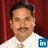Associate Professor at Manipal Institute of Technology em Manipal Institute of Technology
10 de Sep de 2015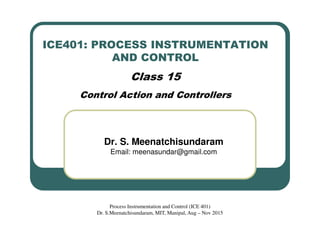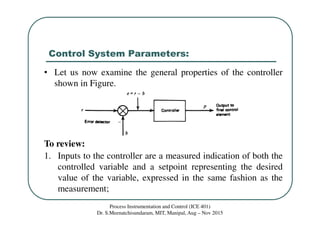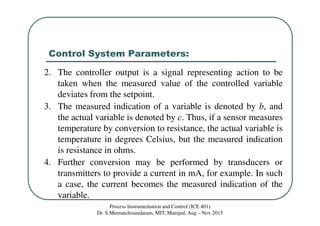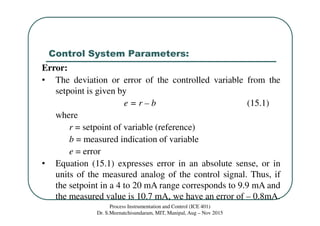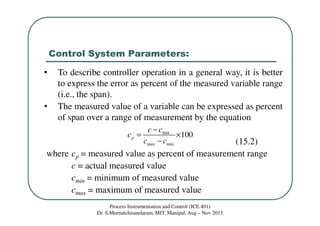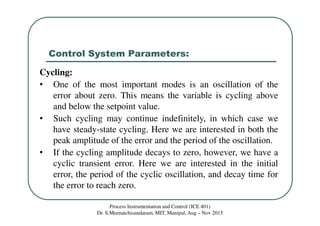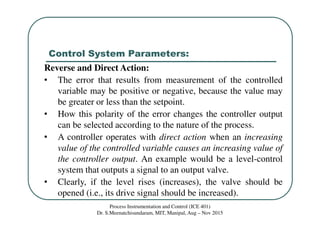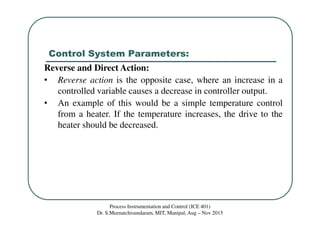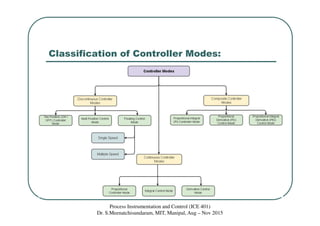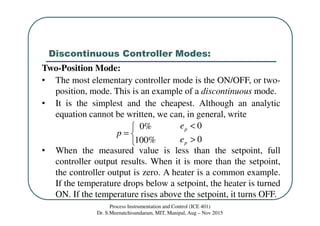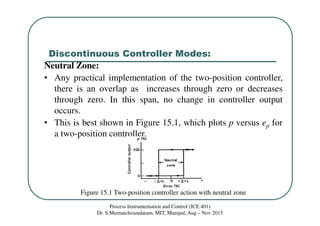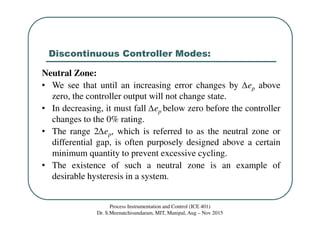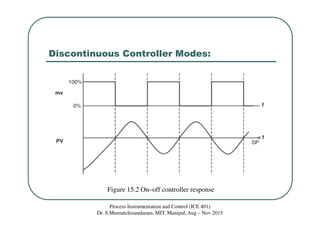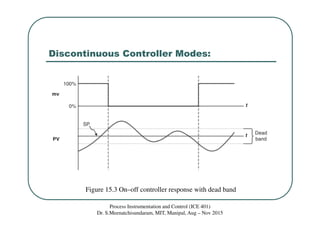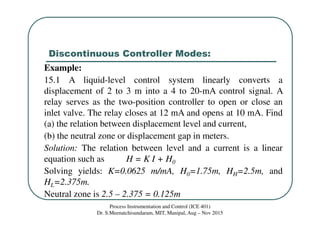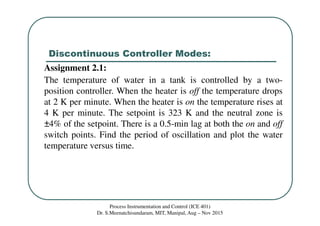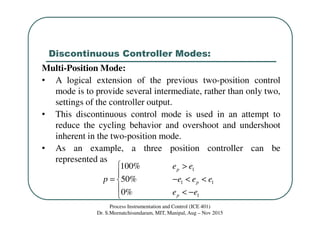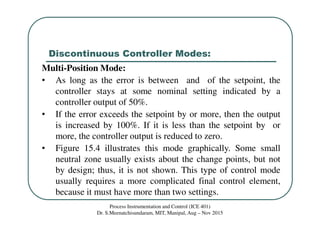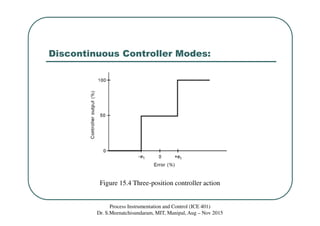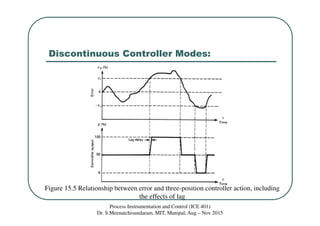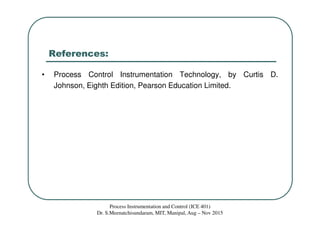1 de 21

### Class 15 control action and controllers

• 1. ICE401: PROCESS INSTRUMENTATION AND CONTROL Class 15 Control Action and Controllers Dr. S. Meenatchisundaram Email: meenasundar@gmail.com Process Instrumentation and Control (ICE 401) Dr. S.Meenatchisundaram, MIT, Manipal, Aug – Nov 2015
• 2. Control System Parameters: Process Instrumentation and Control (ICE 401) Dr. S.Meenatchisundaram, MIT, Manipal, Aug – Nov 2015 • Let us now examine the general properties of the controller shown in Figure. To review: 1. Inputs to the controller are a measured indication of both the controlled variable and a setpoint representing the desired value of the variable, expressed in the same fashion as the measurement;
• 3. Control System Parameters: Process Instrumentation and Control (ICE 401) Dr. S.Meenatchisundaram, MIT, Manipal, Aug – Nov 2015 2. The controller output is a signal representing action to be taken when the measured value of the controlled variable deviates from the setpoint. 3. The measured indication of a variable is denoted by b, and the actual variable is denoted by c. Thus, if a sensor measures temperature by conversion to resistance, the actual variable is temperature in degrees Celsius, but the measured indication is resistance in ohms. 4. Further conversion may be performed by transducers or transmitters to provide a current in mA, for example. In such a case, the current becomes the measured indication of the variable.
• 4. Control System Parameters: Process Instrumentation and Control (ICE 401) Dr. S.Meenatchisundaram, MIT, Manipal, Aug – Nov 2015 Error: • The deviation or error of the controlled variable from the setpoint is given by e = r – b (15.1) where r = setpoint of variable (reference) b = measured indication of variable e = error • Equation (15.1) expresses error in an absolute sense, or in units of the measured analog of the control signal. Thus, if the setpoint in a 4 to 20 mA range corresponds to 9.9 mA and the measured value is 10.7 mA, we have an error of – 0.8mA.
• 5. Control System Parameters: Process Instrumentation and Control (ICE 401) Dr. S.Meenatchisundaram, MIT, Manipal, Aug – Nov 2015 • To describe controller operation in a general way, it is better to express the error as percent of the measured variable range (i.e., the span). • The measured value of a variable can be expressed as percent of span over a range of measurement by the equation (15.2) where cp = measured value as percent of measurement range c = actual measured value cmin = minimum of measured value cmax = maximum of measured value min max min 100p c c c c c − = × −
• 6. Control System Parameters: Process Instrumentation and Control (ICE 401) Dr. S.Meenatchisundaram, MIT, Manipal, Aug – Nov 2015 Cycling: • One of the most important modes is an oscillation of the error about zero. This means the variable is cycling above and below the setpoint value. • Such cycling may continue indefinitely, in which case we have steady-state cycling. Here we are interested in both the peak amplitude of the error and the period of the oscillation. • If the cycling amplitude decays to zero, however, we have a cyclic transient error. Here we are interested in the initial error, the period of the cyclic oscillation, and decay time for the error to reach zero.
• 7. Control System Parameters: Process Instrumentation and Control (ICE 401) Dr. S.Meenatchisundaram, MIT, Manipal, Aug – Nov 2015 Reverse and Direct Action: • The error that results from measurement of the controlled variable may be positive or negative, because the value may be greater or less than the setpoint. • How this polarity of the error changes the controller output can be selected according to the nature of the process. • A controller operates with direct action when an increasing value of the controlled variable causes an increasing value of the controller output. An example would be a level-control system that outputs a signal to an output valve. • Clearly, if the level rises (increases), the valve should be opened (i.e., its drive signal should be increased).
• 8. Control System Parameters: Process Instrumentation and Control (ICE 401) Dr. S.Meenatchisundaram, MIT, Manipal, Aug – Nov 2015 Reverse and Direct Action: • Reverse action is the opposite case, where an increase in a controlled variable causes a decrease in controller output. • An example of this would be a simple temperature control from a heater. If the temperature increases, the drive to the heater should be decreased.
• 9. Classification of Controller Modes: Process Instrumentation and Control (ICE 401) Dr. S.Meenatchisundaram, MIT, Manipal, Aug – Nov 2015
• 10. Discontinuous Controller Modes: Process Instrumentation and Control (ICE 401) Dr. S.Meenatchisundaram, MIT, Manipal, Aug – Nov 2015 Two-Position Mode: • The most elementary controller mode is the ON/OFF, or two- position, mode. This is an example of a discontinuous mode. • It is the simplest and the cheapest. Although an analytic equation cannot be written, we can, in general, write • When the measured value is less than the setpoint, full controller output results. When it is more than the setpoint, the controller output is zero. A heater is a common example. If the temperature drops below a setpoint, the heater is turned ON. If the temperature rises above the setpoint, it turns OFF. 00% 0100% p p e p e < =  >
• 11. Discontinuous Controller Modes: Process Instrumentation and Control (ICE 401) Dr. S.Meenatchisundaram, MIT, Manipal, Aug – Nov 2015 Neutral Zone: • Any practical implementation of the two-position controller, there is an overlap as increases through zero or decreases through zero. In this span, no change in controller output occurs. • This is best shown in Figure 15.1, which plots p versus ep for a two-position controller. Figure 15.1 Two-position controller action with neutral zone
• 12. Discontinuous Controller Modes: Process Instrumentation and Control (ICE 401) Dr. S.Meenatchisundaram, MIT, Manipal, Aug – Nov 2015 Neutral Zone: • We see that until an increasing error changes by ∆ep above zero, the controller output will not change state. • In decreasing, it must fall ∆ep below zero before the controller changes to the 0% rating. • The range 2∆ep, which is referred to as the neutral zone or differential gap, is often purposely designed above a certain minimum quantity to prevent excessive cycling. • The existence of such a neutral zone is an example of desirable hysteresis in a system.
• 13. Discontinuous Controller Modes: Process Instrumentation and Control (ICE 401) Dr. S.Meenatchisundaram, MIT, Manipal, Aug – Nov 2015 Figure 15.2 On–off controller response
• 14. Discontinuous Controller Modes: Process Instrumentation and Control (ICE 401) Dr. S.Meenatchisundaram, MIT, Manipal, Aug – Nov 2015 Figure 15.3 On–off controller response with dead band
• 15. Discontinuous Controller Modes: Process Instrumentation and Control (ICE 401) Dr. S.Meenatchisundaram, MIT, Manipal, Aug – Nov 2015 Example: 15.1 A liquid-level control system linearly converts a displacement of 2 to 3 m into a 4 to 20-mA control signal. A relay serves as the two-position controller to open or close an inlet valve. The relay closes at 12 mA and opens at 10 mA. Find (a) the relation between displacement level and current, (b) the neutral zone or displacement gap in meters. Solution: The relation between level and a current is a linear equation such as H = K I + H0 Solving yields: K=0.0625 m/mA, H0=1.75m, HH=2.5m, and HL=2.375m. Neutral zone is 2.5 – 2.375 = 0.125m
• 16. Discontinuous Controller Modes: Process Instrumentation and Control (ICE 401) Dr. S.Meenatchisundaram, MIT, Manipal, Aug – Nov 2015 Assignment 2.1: The temperature of water in a tank is controlled by a two- position controller. When the heater is off the temperature drops at 2 K per minute. When the heater is on the temperature rises at 4 K per minute. The setpoint is 323 K and the neutral zone is ±4% of the setpoint. There is a 0.5-min lag at both the on and off switch points. Find the period of oscillation and plot the water temperature versus time.
• 17. Discontinuous Controller Modes: Process Instrumentation and Control (ICE 401) Dr. S.Meenatchisundaram, MIT, Manipal, Aug – Nov 2015 Multi-Position Mode: • A logical extension of the previous two-position control mode is to provide several intermediate, rather than only two, settings of the controller output. • This discontinuous control mode is used in an attempt to reduce the cycling behavior and overshoot and undershoot inherent in the two-position mode. • As an example, a three position controller can be represented as 1 1 1 1 100% 50% 0% p p p e e p e e e e e  >  = − < <  < −
• 18. Discontinuous Controller Modes: Process Instrumentation and Control (ICE 401) Dr. S.Meenatchisundaram, MIT, Manipal, Aug – Nov 2015 Multi-Position Mode: • As long as the error is between and of the setpoint, the controller stays at some nominal setting indicated by a controller output of 50%. • If the error exceeds the setpoint by or more, then the output is increased by 100%. If it is less than the setpoint by or more, the controller output is reduced to zero. • Figure 15.4 illustrates this mode graphically. Some small neutral zone usually exists about the change points, but not by design; thus, it is not shown. This type of control mode usually requires a more complicated final control element, because it must have more than two settings.
• 19. Discontinuous Controller Modes: Process Instrumentation and Control (ICE 401) Dr. S.Meenatchisundaram, MIT, Manipal, Aug – Nov 2015 Figure 15.4 Three-position controller action
• 20. Discontinuous Controller Modes: Process Instrumentation and Control (ICE 401) Dr. S.Meenatchisundaram, MIT, Manipal, Aug – Nov 2015 Figure 15.5 Relationship between error and three-position controller action, including the effects of lag
• 21. References: • Process Control Instrumentation Technology, by Curtis D. Johnson, Eighth Edition, Pearson Education Limited. Process Instrumentation and Control (ICE 401) Dr. S.Meenatchisundaram, MIT, Manipal, Aug – Nov 2015